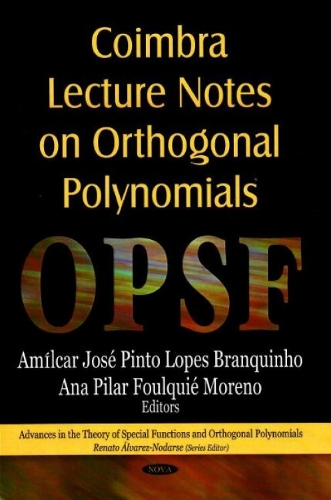•# Coimbra Lecture Notes on Orthogonal Polynomials (Hardback)

(editor), (editor)
£224.99
Hardback 233 Pages / Published: 01/02/2008
• We can order this

Usually dispatched within 3 weeks

Orthogonal Polynomials and Special Functions (OPSF) have a very rich history, going back to 19th century when mathematicians and physicists tried to solve the most important deferential equations of mathematical physics. Hermite-Pade approximation was also introduced at that time, to prove the transcendence of the remarkable constant e (the basis of the natural logarithm). Since then OPSF has developed to a standard subject within mathematics, which is driven by applications. The applications are numerous, both within mathematics (e.g. statistics, combinatory, harmonic analysis, number theory) and other sciences, such as physics, biology, computer science, chemistry. The main reason for the fact that OPSF has been so successful over the centuries is its usefulness in other branches of mathematics and physics, as well as other sciences. There are many different aspects of OPSF. Some of the most important developments for OPSF are related to the theory of rational approximation of analytic functions, in particular the extension to simultaneous rational approximation to a system of functions. Important tools for rational approximation are Riemann-Hilbert problems, the theory of orthogonal polynomials, logarithmic potential theory, and operator theory for difference operators. This new book presents the latest research in the field.

Publisher: Nova Science Publishers Inc
ISBN: 9781600219726
Number of pages: 233
Weight: 668 g
Dimensions: 180 x 260 mm